# Repeated Measures ANOVA with Huynh and Feldt Epsilon Correction

The test statistics is: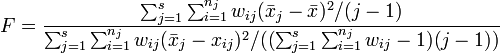$F=\frac{\sum^s_{j=1} \sum^{n_j}_{i = 1} w_{ij}(\bar{x}_j - \bar{x})^2 / (j-1)} {\sum^s_{j=1} \sum^{n_j}_{i=1} w_{ij}(\bar{x}_j - x_{ij})^2 / ((\sum^s_{j=1} \sum^{n_j}_{i = 1} w_{ij}-1) (j - 1))}$

where: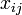$x_{ij}$ is the value of the$i$th of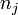$n_j$ observations in the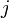$j$th of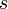$s$ groups, where$x_{ij}$ has been 'centered' such that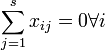$\sum^s_{j=1} x_{ij} = 0\forall i$,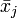$\bar{x}_j$ is the average in the$j$th group,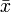$\bar{x}$ is the overall average,$w_{ij}$ is the calibrated weight,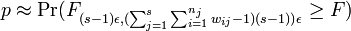$p \approx \Pr(F_{(s-1)\epsilon,(\sum^s_{j=1} \sum^{n_j}_{i = 1} w_{ij}-1) (s - 1))\epsilon} \ge F )$, and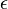$\epsilon$ is computed using the Greenhouse-Geisser method.

and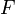$F$ is evaulated using the F-distribution with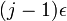$(j-1)\epsilon$ and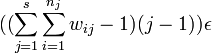$((\sum^s_{j=1} \sum^{n_j}_{i = 1} w_{ij}-1) (j - 1))\epsilon$ degrees of freedom, where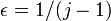$\epsilon = 1 / (j - 1)$.